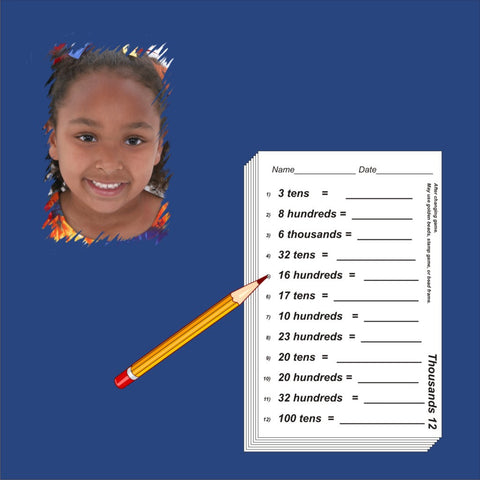## Discounted Third Grade Blackline Set

• 38000

• Second grade blacklines for reinforcement
• Photocopy for written work. Also includes answer sheets
• Numerous exercise sets

Thousand Line & Labels, Order (blue), Order (pink), Order (green), Place Value (blue), Place Value (pink), Place Value (green), Numeration (blue), More Numeration (pink), Tens (gold), Money B, Time B, Problem Solving B, Mixed Practice B, Thousands (green), Problem Solving C, Mixed Practice C, Geometry Introduction, Money C, Time C, Fraction Match, Roman Numerals, Problem Solving D, Mixed Practice D, Fraction Concepts, Decimal Introduction